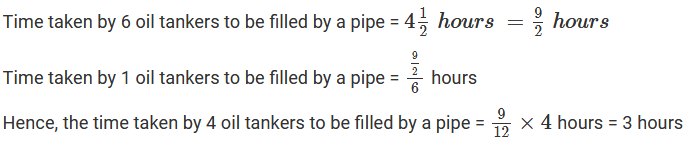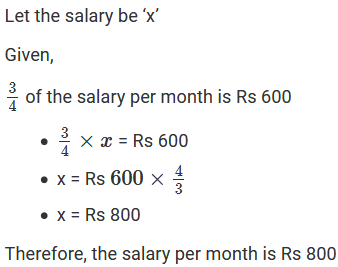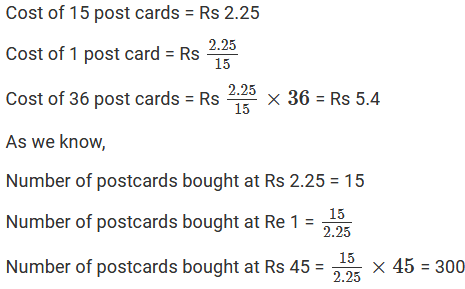×#### Thank you for registering.

One of our academic counsellors will contact you within 1 working day.

Click to Chat

1800-1023-196

+91-120-4616500

CART 0

• 0

MY CART (5)

Use Coupon: CART20 and get 20% off on all online Study Material

ITEM
DETAILS
MRP
DISCOUNT
FINAL PRICE
Total Price: Rs.

There are no items in this cart.
Continue Shopping• Complete JEE Main/Advanced Course and Test Series
• OFFERED PRICE: Rs. 15,900
• View Details

```Chapter 10: Unitary Method Exercise – 10.1

Question: 1

20 chocolates cost Rs 320. Find the cost of 35 such chocolates.

Solution:

Cost of 20 chocolates = Rs 320

Cost of 1 chocolate = Rs (320/20)

Therefore, the cost of 35 chocolates = Rs ((320/20) × 35) = Rs 560

Question: 2

The cost of 40 meters of cloth is Rs 200. Find the cost of 50 meters of cloth.

Solution:

Cost of 40 meters of cloth = Rs 200

Cost of 1 meter of cloth = Rs (200/40)

Therefore, the cost of 50 chocolates = Rs ((200/40) × 50) = Rs 250

Question: 3

A car can cover a distance of 522 km on 36 liters of petrol. How far can it travel on 14 litres of petrol?

Solution:

Number of kilometers a car can cover by using 36 liters = 522 km

Number of kilometers a car can cover by using 1 liter = 522/36 km

Hence, the number of kilometers a car can cover by using 14 liters = ((522/36) × 14) km = 203 km

Question: 4

Travelling 900 km by rail costs Rs 280. What would be the fare for a journey of 360 km when a person travels by the same class?

Solution:

Cost of travelling 900 km by rail = Rs 280

Cost of travelling 1 km by rail = Rs (280/900)

Hence, Cost of travelling 360 km by rail = Rs (280/900) × 360 = Rs 112

Question: 5

If 6 oil tankers can be filled by a pipe in 4(1/2) hours, how long does the pipe take to fill 4 such oil tankers?

Solution:Question: 6

3/4 of the salary per month is Rs 600. What is the salary per month?

Solution:Question: 7

The cost of 32 tables is Rs 23520. Find the number of such tables that can be purchased for Rs 51450.

Solution:

Number of tables bought for Rs 23520 = 32

Number of tables bought for Re 1 = 32/23520

Number of tables bought for Rs 51450 = (32/23520) × 51450 = 70

Question: 8

The yield of wheat from 6 hectares is 280 quintals. Find the number of hectares required for a yield of 225 quintals.

Solution:

Number of hectares required for a yield of 280 quintals = 6 hectares

Number of hectares required for a yield of 1 quintal = 6/280 hectares

Hence, the number of hectares required for a yield of 225 quintals = 6/280 × 225 hectares = 4(23/28) hectares

Question: 9

Fifteen post cards cost Rs 2.25. What will be the cost of 36 post cards? How many postcards can we buy in Rs 45?

Solution:Question: 10

A rail journey of 75 km costs Rs 215. How much will a journey of 120 km cost?

Solution:

Cost of a rail journey of 75 km = Rs 215

Cost of a rail journey of 1 km = Rs 215/75

Cost of a rail journey of 120 km = Rs 215/75 × 120 = Rs 344

Question: 11

If the sales tax on a purchase worth Rs 60 is Rs 4.20. What will be the sales tax on the purchase worth of Rs 150?

Solution:

Sales tax on the purchase worth of Rs 60 = Rs 4.20

Sales tax on the purchase worth of Re 1 = Rs 4.2060

Sales tax on the purchase worth of Rs 150 = Rs (4.20/60) × 150 = Rs 10.50

Question: 12

52 packets of 12 pencils each, cost Rs 499.20. Find the cost of 65 packets of 10 pencils each.

Solution:

Total number of pencils in 52 packets of 12 pencils each = 52 × 12 = 624

Cost of 624 pencils = Rs 499.20

Cost of 1 pencil = Rs 499.20/624

Now,

Number of pencils in 65 packets of 10 pencils each = 65 x 10 = 650 pencils

Therefore, cost of 650 pencils = Rs (499.20/624) × 650 = Rs 520.
```### Course Features

• 728 Video Lectures
• Revision Notes
• Previous Year Papers
• Mind Map
• Study Planner
• NCERT Solutions
• Discussion Forum
• Test paper with Video Solution